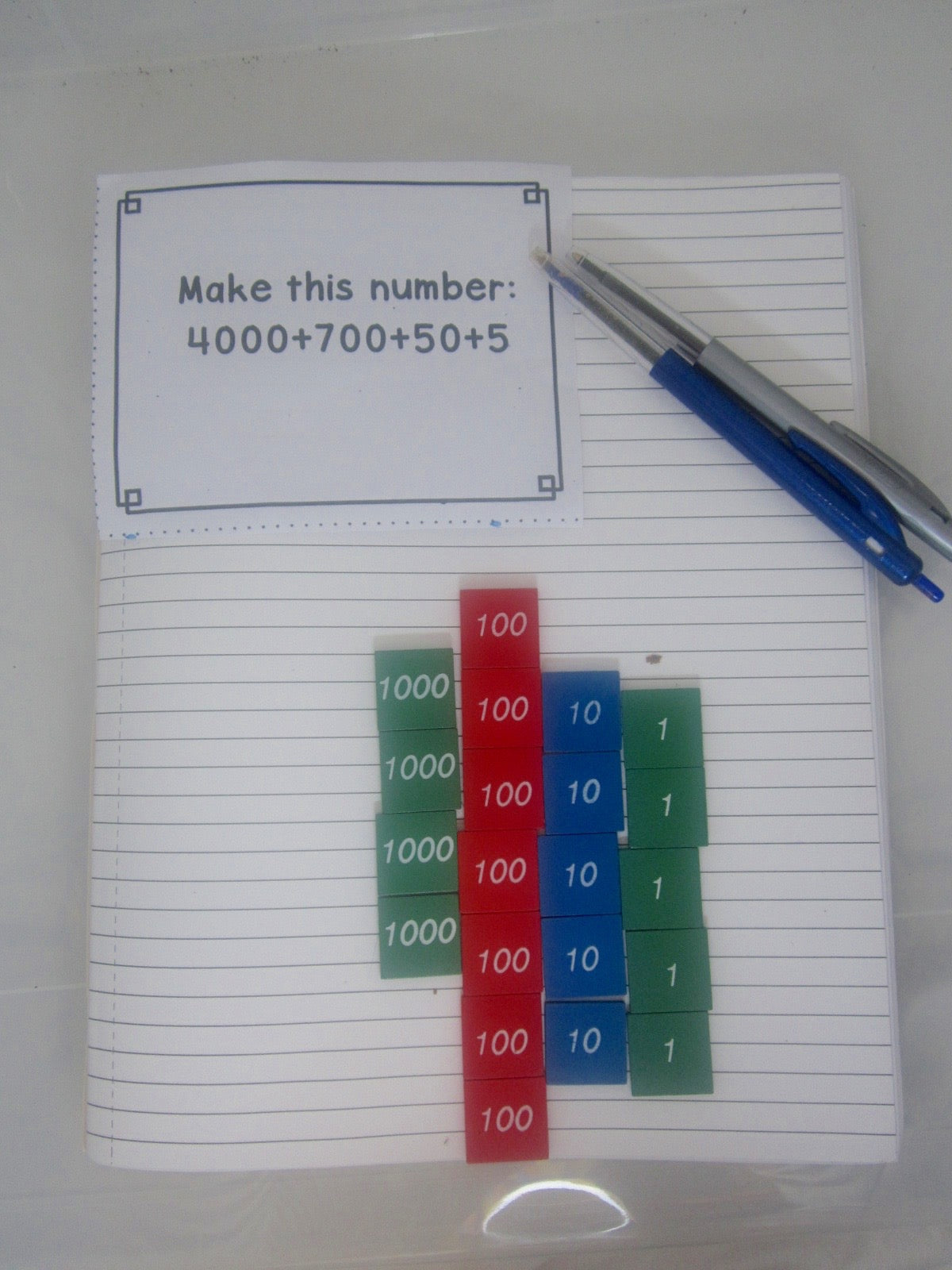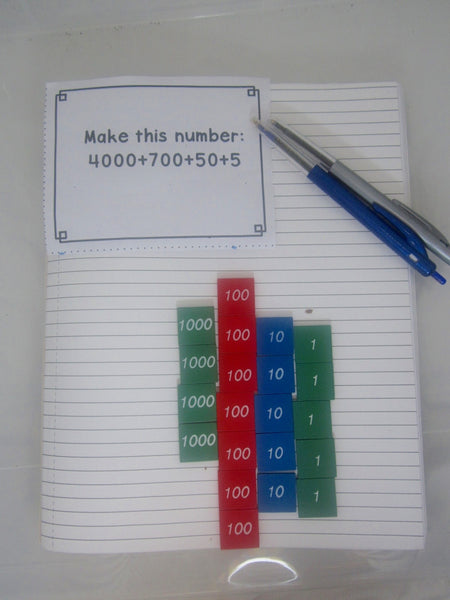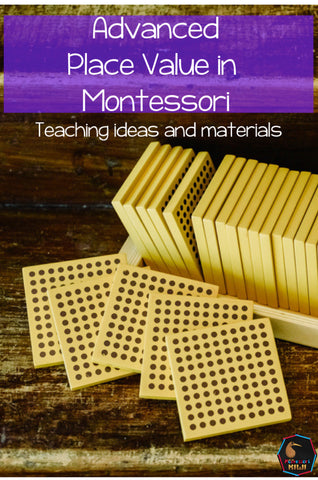Our Montessori equipment is great for teaching place value.

In Montessori we start quite early with 'big' numbers for example it is not uncommon for a 6 year old to be adding two four digit numbers together. However students aren't always able to articulate the place value of what they are adding. I'll give you an example if I gave the equation

4567

+2321

--------

to a student  and asked them what they were doing they would probably say 7 + 1 for the units, then 6 + 2 for the tens, then 5 plus 3 for the hundreds. This is different to saying 60 + 20 for the tens or 500  + 300 for the hundreds

Simply put value is about understanding the meaning of a certain number. In the number 4325 the 3 is in the hundreds place so its value is 300.

Place Value also has other underlying concepts for example

- Is the student aware of embedded place value? For example 490 is 49 groups of ten or the number for 32 groups of ten is 320.

- Is the student aware of how a number such as 3489 can be broken down into its place value amounts e.g. 3000 + 400 + 80 + 9

- Is the student able to use place value to see patterns, for example 100 more than 2534 is 2634. Another 100 is 2734 and so on.

When could you teach this?

• This works best when naturally integrated alongside equipment. For example when you are giving a child an equation stop and ask them what the number means.
• If this isn't practical when you are conferencing with a student you could talk to them then about it.
• Having task cards with specific questions on them is also helpful. Students could alternate between traditional equation card questions and place value questions

My advanced place value task cards have questions like those above to help students learn these concepts.

They work best with the stamp game or with the golden beads. However you can use them with any place value equipment you have.Get a free sample of these cards by clicking HERETo find out more about the progress of children's thinking with math strategies see this blog post that explains how children think about maths.

or find out more about teaching place value here